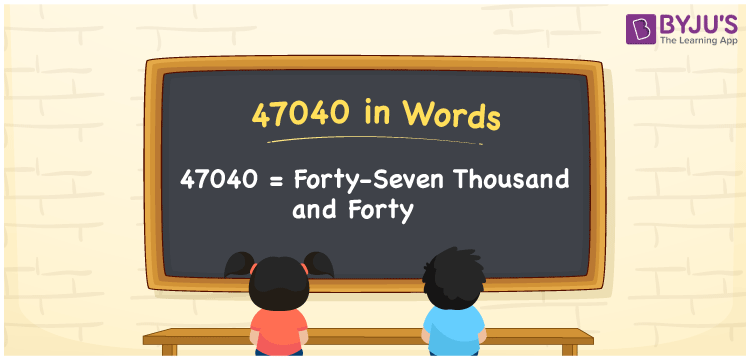# 47040 in Words

47040 in words is Forty-Seven Thousand and Forty. For example, if you buy a watch worth Rs. 47040, then you can say, “I bought a watch worth Rupees Forty-Seven Thousand and Forty”. 47040 is a cardinal number since it represents a specific quantity or value of something. We can convert the number 47040 in words easily using a place value table. This article provides a complete explanation of how to convert the number 47040 into words with the help of a place value chart.

 47040 in Words Forty-Seven Thousand and Forty Forty-Seven Thousand and Forty in numerical form 47040

## 47040 in English Words

Generally, with the help of the English alphabet, we write numbers in words. Therefore, the number 47040 in English can be written as Forty-Seven Thousand and Forty.## How to Write 47040 in Words?

The number 47040 can be converted into words quickly and easily using a place value table. Since 47040 is a five-digit number. Hence, we need a table of 5 columns to depict its place value chart. This can be done as below.

 Ten Thousands Thousands Hundreds Tens Ones 4 7 0 4 0

Hence, we can write the expanded form as:

4 x Ten Thousand + 7 x Thousand + 0 x Hundred + 4 x Ten + 0 x One

= 4 x 10000 + 7 x 1000 + 0 x 100 + 4 x 10 + 0 x 1

= 40000 + 7000 + 0 + 40 + 0

= 40000 + 7000 + 40

= 47040

= Forty-Seven Thousand and Forty

Therefore, 47040 in words is written as Forty-Seven Thousand and Forty

Interesting way of writing 47040 in words

4 = Four

47 = Forty-Seven

470 = Four Hundred and Seventy

4704 = Four Thousand Seven Hundred Four

47040 = Forty-Seven Thousand and Forty

Thus, the word form of the number 47040 is Forty-Seven Thousand and Forty

47040 is a natural number that is the successor of 47039 and the predecessor of 47041

• 47040 in words – Forty-Seven Thousand and Forty
• Is 47040 an odd number? – No
• Is 47040 an even number? – Yes
• Is 47040 a perfect square number? – No
• Is 47040 a perfect cube number? – No
• Is 47040 a prime number? – No
• Is 47040 a composite number? – Yes

## Frequently Asked Questions on 47040 in Words

Q1

### How do you write 47040 in words?

47040 in words is written as Forty-Seven Thousand and Forty.
Q2

### Simplify 40000 + 7040, and express in words.

Simplifying 40000 + 7040, we get 47040. Therefore, the number 47040 in words is Forty-Seven Thousand and Forty.
Q3

### What is the place value of 7 in 47040?

The place value of 7 in 47040 is Thousands.